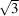International Tables for Crystallography (2006). Vol. C. ch. 1.3, pp. 10-14https://doi.org/10.1107/97809553602060000574

## Contents

• 1.3. Twinning  (pp. 10-14)
• 1.3.1. General remarks  (p. 10) | html | pdf |
• 1.3.2. Twin lattices  (pp. 10-12) | html | pdf |
• 1.3.2.1. Examples  (pp. 11-12) | html | pdf |
• 1.3.3. Implication of twinning in reciprocal space  (p. 12) | html | pdf |
• 1.3.4. Twinning by merohedry  (pp. 12-14) | html | pdf |
• 1.3.5. Calculation of the twin element  (p. 14) | html | pdf |
• References | html | pdf |
• Figures
• Fig. 1.3.2.1. ( a ) Projection of the lattices of the twin components of a monoclinic twinned crystal (unique axis c , γ = 93°) with twin index 3  (p. 11) | html | pdf |
• Fig. 1.3.2.2. Projection of the lattices of the twin components of an orthorhombic twinned crystal ( oP , b =a ) with twin index 2  (p. 12) | html | pdf |
• Fig. 1.3.2.3. Projection of the lattices of the twin components of an orthorhombic twinned crystal ( oC , b = 2 a ) with twin index 4  (p. 12) | html | pdf |
• Tables
• Table 1.3.2.1. Lattice planes and rows that are perpendicular to each other independently of the metrical parameters  (p. 11) | html | pdf |
• Table 1.3.4.1. Possible twin operations for twins by merohedry  (p. 13) | html | pdf |
• Table 1.3.4.2. Simulated Laue classes, extinction symbols, simulated `possible space groups', and possible true space groups for crystals twinned by merohedry (type 2)  (p. 13) | html | pdf |